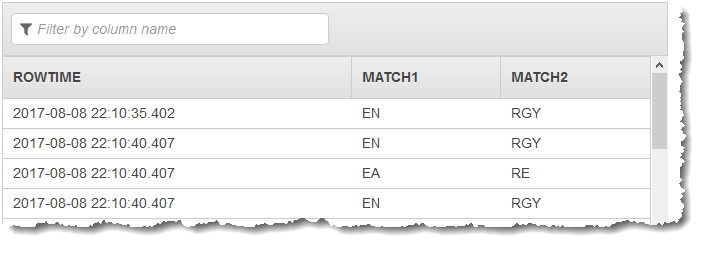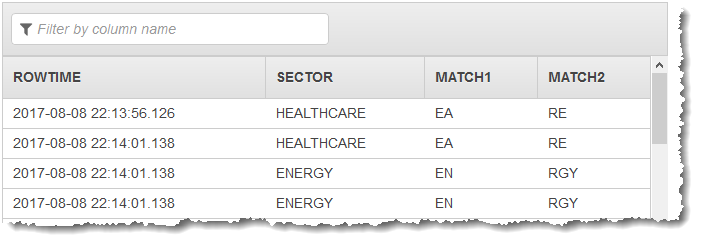REGEX_LOG_PARSE - Amazon Kinesis Data Analytics
AWS 文档中描述的 AWS 服务或功能可能因区域而异。要查看适用于中国区域的差异，请参阅中国的 AWS 服务入门

# REGEX_LOG_PARSE

``REGEX_LOG_PARSE (<character-expression>,<regex-pattern>,<columns>)<regex-pattern> := <character-expression>[OBJECT] <columns> := <columnname> [ <datatype> ] {, <columnname> <datatype> }*``

## 示例

### 示例数据集

``````
(ticker_symbol  VARCHAR(4),
sector          VARCHAR(16),
change          REAL,
price           REAL)
``````

### 示例 1：从两个捕获组返回结果

``````CREATE OR REPLACE STREAM "DESTINATION_SQL_STREAM" (match1 VARCHAR(1024), match2 VARCHAR(1024));

CREATE OR REPLACE PUMP "STREAM_PUMP" AS INSERT INTO "DESTINATION_SQL_STREAM"
SELECT STREAM T.REC.COLUMN1, T.REC.COLUMN2
FROM
(SELECT STREAM SECTOR,
REGEX_LOG_PARSE(SECTOR, '.*([E].).*([R].*)') AS REC
FROM SOURCE_SQL_STREAM_001) AS T;
``````### 示例 2：从两个捕获组返回一个流字段和结果

``````CREATE OR REPLACE STREAM "DESTINATION_SQL_STREAM" (sector VARCHAR(24), match1 VARCHAR(24), match2 VARCHAR(24));

CREATE OR REPLACE PUMP "STREAM_PUMP" AS INSERT INTO "DESTINATION_SQL_STREAM"
SELECT STREAM T.SECTOR, T.REC.COLUMN1, T.REC.COLUMN2
FROM
(SELECT STREAM SECTOR,
REGEX_LOG_PARSE(SECTOR, '.*([E].).*([R].*)') AS REC
FROM SOURCE_SQL_STREAM_001) AS T;
``````## 快速 Regex 引用

 [xyz] 查找 x、y 或 z 的其中一个字符 [^abc] 查找除 x、y 或 z 之外的任意单一字符 [r-z] 查找介于 r 和 z 之间的任意单一字符 [r-zR-Z] 查找介于 r 和 z 之间或介于 R 和 Z 之间的任意单一字符 ^ 行首 \$ 行尾 \A 字符串开始 \z 字符串末尾 。任意单一字符 \s 查找任意空格字符 \S 查找任意非空格字符 \d 查找任意数字 \D 查找任意非数字 \w 查找任意单词字符 (字母、数字、下划线) \W 查找任意非单词字符 \b 查找任意字边界 (...) 捕获包含的所有内容 (x|y) 查找 x 或 y (也适用于 \d 或 \s 等符号) x? 查找零个或一个 x (也适用于 \d 或 \s 等符号) x* 查找零个或零个以上的 x (也适用于 \d 或 \s 等符号) x+ 查找一个或多个 x (也适用于 \d 或 \s 等符号) x{3} 精确查找 3 个 x (也适用于 \d 或 \s 等符号) x{3,} 查找 3 个或更多 x (也适用于 \d 或 \s 等符号) x{3,6} 查找 3 至 6 个 x (也适用于 \d 或 \s 等符号)